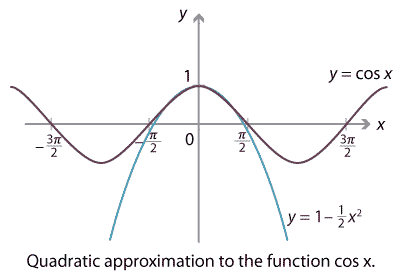### Approximating functions

If we are given a function $$f(x)$$, we can try to find a polynomial $$p(x)$$ which approximates that function. Indeed, if we know some of the values of $$f$$,

$f(x_1) = y_1, \qquad f(x_2) = y_2, \qquad \dots, \qquad f(x_n) = y_n,$

then we can find a polynomial $$p(x)$$ which takes those values, as we just saw.

That is one type of approximation. The following example shows another type of approximation: finding a polynomial which agrees with $$f$$, and its derivatives, at a point.

#### Example

Find a quadratic polynomial $$p(x)$$ which approximates the function $$f(x) = \cos x$$ in the sense that $$p$$ agrees with $$f$$ in value, and in its first two derivatives, at $$x=0$$, i.e.,

$p(0) = f(0), \qquad p'(0) = f'(0) \qquad \text{and} \qquad p''(0) = f''(0).$

(Reality check: We're asked to find a quadratic polynomial satisfying three conditions. This makes sense since a quadratic has three coefficients.)

#### Solution

First of all we can compute $$f'(x) = -\sin x$$ and $$f''(x) = -\cos x$$, so $$f(0) = 1$$, $$f'(0) = 0$$ and $$f''(0) = -1$$. Thus we want to find a quadratic polynomial $$p(x)$$ such that

$p(0) = 1, \qquad p'(0) = 0 \qquad \text{and} \qquad p''(0) = -1.$

Let $$p(x) = ax^2 + bx + c$$, where $$a,b,c$$ are real coefficients. Then we have $$p'(x) = 2ax + b$$ and $$p''(x) = 2a$$, so the three equations above become

$c = 1, \qquad b = 0 \qquad \text{and} \qquad 2a = -1.$

Hence $$p(x) = -\frac{1}{2} x^2 + 1$$.

We just showed that $$p(x) = 1 - \frac{1}{2} x^2$$ is a good approximation to $$f(x) = \cos x$$ near $$x=0$$. A comparison of the graphs shows how good the approximation is.Detailed description of diagram

If we continue on, we can find a cubic approximation to $$\cos x$$ which agrees up to the third derivative at $$x=0$$, a quartic which agrees up to the fourth derivative, and so on. In this way we get a sequence of polynomials which approximate $$\cos x$$ increasingly better.

$\begin{array}{rclcl} \cos x & \sim & 1 & & \text{'constant' approximation} \\[2.5ex] &\sim & 1 - \dfrac{x^2}{2!} & & \text{quadratic approximation} \\[2.5ex] & \sim & 1 - \dfrac{x^2}{2!} + \dfrac{x^4}{4!} & & \text{quartic approximation} \\[2.5ex] & \sim & 1 - \dfrac{x^2}{2!} + \dfrac{x^4}{4!} - \dfrac{x^6}{6!} & & \text{degree-6 approximation} \end{array}$

Continuing these terms on to infinity results in an infinite series approximating $$\cos x$$. In fact this infinite series equals $$\cos x$$:

$\cos x = 1 - \frac{x^2}{2!} + \frac{x^4}{4!} - \frac{x^6}{6!} + \frac{x^8}{8!} - \dots = \sum_{k=0}^\infty (-1)^k \frac{x^{2k}}{(2k)!}.$

A series like this is known as a power series. Many functions can be well approximated by power series. Some are approximated completely and 'eventually exactly' like this one. For others, the power series only gives a good approximation in an interval of convergence. This topic is studied extensively in university science and engineering courses.

Next page - Links forward - Sums and products of roots